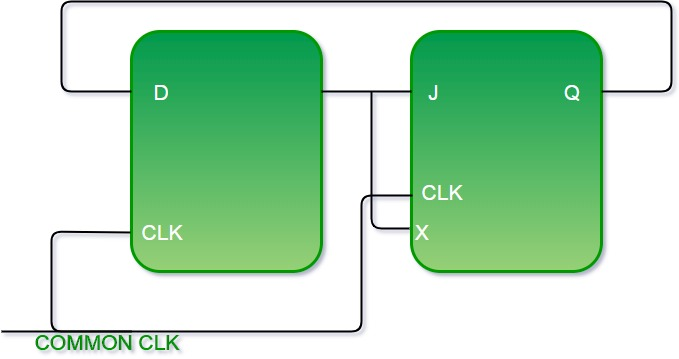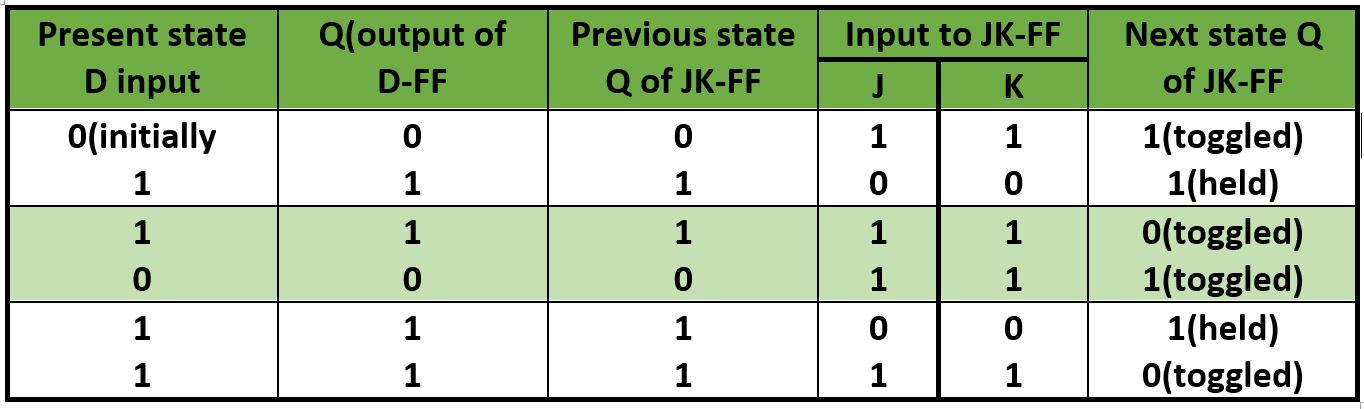# GATE | GATE-CS-2015 (Set 1) | Question 47

A positive edge-triggered D flip-flop is connected to a positive edge-triggered JK flipflop as follows. The Q output of the D flip-flop is connected to both the J and K inputs of the JK flip-flop, while the Q output of the JK flip-flop is connected to the input of the D flip-flop. Initially, the output of the D flip-flop is set to logic one and the output of the JK flip-flop is cleared. Which one of the following is the bit sequence (including the initial state) generated at the Q output of the JK flip-flop when the flip-flops are connected to a free-running common clock? Assume that J = K = 1 is the toggle mode and J = K = 0 is the state-holding mode of the JK flip-flop. Both the flip-flops have non-zero propagation delays.
(A) 0110110…
(B) 0100100…
(C) 011101110…
(D) 011001100…

Explanation:Initially Q output of D – FF  = 1

Initially Q output of JK – FF  = 0

Now with the help of present state and next state table we can see what is happening in circuit.

• Toggle: J = K = 1
• Hold : J = K = 0We see from table Q output of D-FF is going to next state input of JK-FF and the bits sequence produced is like 110110…..

Including initial condition (0) we get output as 0110110110….

Another Explanation:

Here, it is given that JK flip flop will toggle when J = K = 1 and it will retain the output if J = K = 0.
Also, the output of the D flip flop would remain the same as the input.

So, we have

Initial output : D = 1

JK = 0

```After clock 1 : D = 0 (D gets 0 as input
from initial output of JK, so output = 0)```

JK = 1 (J = K = 1 from initial output of D, so output would be toggled from 0 to 1)

```After clock 2 : D = 1 (D gets 1 as input
from previous output of JK, so output = 1)```

JK = 1 (J = K = 0 from previous output of D, so output would be retained to 1)

```After clock 3 : D = 1 (D gets 1 as input
from previous output of JK, so output = 1)```

JK = 0 (J = K = 1 from previous output of D, so output would be toggled from 1 to 0)

```After clock 4 : D = 0 (D gets 0 as input
from previous output of JK, so output = 0)```

JK = 1 (J = K = 1 from previous output of D, so output would be toggled from 0 to 1)

```After clock 5 : D = 1 (D gets 1 as input
from previous output of JK, so output = 1)```

JK = 1 (J = K = 0 from previous output of D, so output would be retained to 1)

Thus, the bit sequence generated at the Q output of the JK flip flop will be 0110110…

My Personal Notes arrow_drop_up
Article Tags :

Be the First to upvote.

Please write to us at contribute@geeksforgeeks.org to report any issue with the above content.